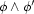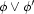Encode Method

One we have created a DomainProduct object O, we can use it to turn a specification into a set of integers encoding the tuples corresponding to this specification. We want to allow the user to write specifications with the following abstract syntax:A tuple satisfies specificationif it contains value. It satisfiesif it satisfies bothand. It satisfiesif it satisfies eitheror.

Instead of explicit connectives, we will simply allow a specification to consist of arbitrarily nested lists, eventually bottoming out with domain values. The outer level is interpreted disjunctively, and each nesting switches the interpretation of the connective: thus the 2nd level is interpreted conjunctively, the 3rd disjunctively, etc. For example, let us consider agreement information limited to just gender and person. The specification:

[[masc [1 3]] [fem 2]]

denotes the 3 tuples [masc 1], [masc 3], [fem 2]. However:

[[masc 1 3] [fem 2]]

just denotes [fem 2] since a tuple cannot contain both 1 and 2. The spec:

[[masc 1] fem]

denotes the 4 tuples [masc 1], [fem 1], [fem 2] and [fem 3].

meth encode(Desc $) {self Disj(Desc$)}
end
meth Disj(Desc $) case Desc of _|then {FoldL Desc fun {$ Accu Desc}
{FS.union Accu
{self Conj(Desc $)}} end self.empty} [] nil then self.empty else @value2set.Desc end end meth Conj(Desc$)
case Desc
of _|then {FoldL Desc
fun {$Accu Desc} {FS.intersect Accu {self Disj(Desc$)}}
end self.full}
[] nil then self.full
else @value2set.Desc end
end

Denys Duchier
Version 1.2.0 (20010221)